Differential Equations and Computational Simulations III
Electron. J. Diff. Eqns., Conf. 01, 1997, pp. 119, 127.

### A global solution curve for a class of semilinear equations Philip Korman

Abstract:
We use bifurcation theory to give a simple proof of existence and uniqueness of a positive solution for the problem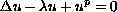for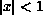, u = 0 on |x| = 1,
where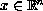, for any positive integer n, and real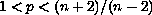,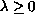. Moreover, we show that all solutions lie on a unique smooth curve of solutions, and all solutions are non-singular. In the process we prove the following assertion, which appears to be of independent interest: the Morse index of the positive solution of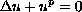for, u = 0 on |x| = 1
is one, for any.

Published November 12, 1998.
Mathematics Subject Classifications: 35J60.
Key words and phrases: Uniqueness of positive solution, Morse index.

Show me the PDF file (121K), TEX file, and other files for this article.

Philip Korman
Institute for Dynamics and
Department of Mathematical Sciences
University of Cincinnati
Cincinnati Ohio 45221-0025 USA